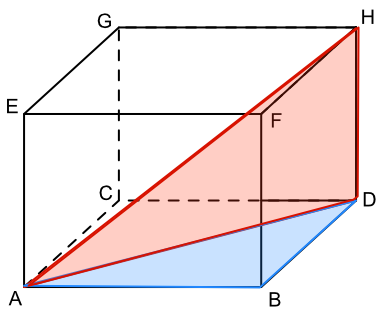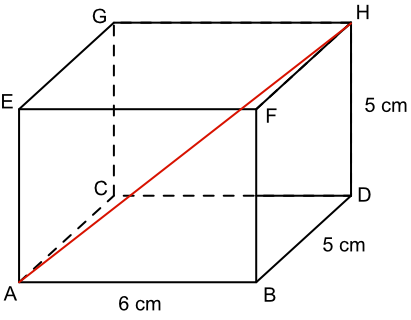Three Dimensional Figures

# Three Dimensional Figures

GCSE(H),

Most trigonometry problems in three dimensions will involve Pythagoras.

Solving the problems involve analysing individual planes, or flat surfaces, to build up a 3D answer.

In the cuboid below, a diagonal has been drawn on the base of the cuboid from point A to point D. This is the hypotenuse of triangle ABD.

The isometric view distorts the angles of the triangles. Note that ABD is a right angle, and that ADH is also a right angle.The diagonal from point A to point H is on a vertical plane which runs across the cuboid: part of the plane is shown by the triangle ADH. The line AD is now one of the shorter sides that forms part of the triangle ADH.

The length of the diagonal AH can be calculated by working out the length AD on triangle ABD, then working out the length AH on triangle ADH.

## Examples

1. What is the length of the diagonal, AH, in this cuboid?Work out the length of the diagonal AD using AB and BD

(AD)^2 = (AB)^2 + (BD)^2

(AD)^2 = 6^2 + 5^2

(AD)^2 = 61

AD = 7.810

Work out the length of the diagonal AH using AD and HD

(AH)^2 = (AD)^2 + (HD)^2

(AH)^2 = 7.810^2 + 5^2

(AH)^2 = 61 + 25

AH = 9.274 (3dp)

Note that (AH)^2 = (AB)^2 + (BD)^2 + (DH)^2

2. Using the cuboid, above, determine the size of the angle X that the diagonal AH makes with the bottom plane of the box ABCD.

With Sin: sin(x) = frac(opposite)(hypotenuse)
sin(x) = frac(5)(7.81)
sin(x) = 0.640
x = sin^-1(0.640) = 39.807º